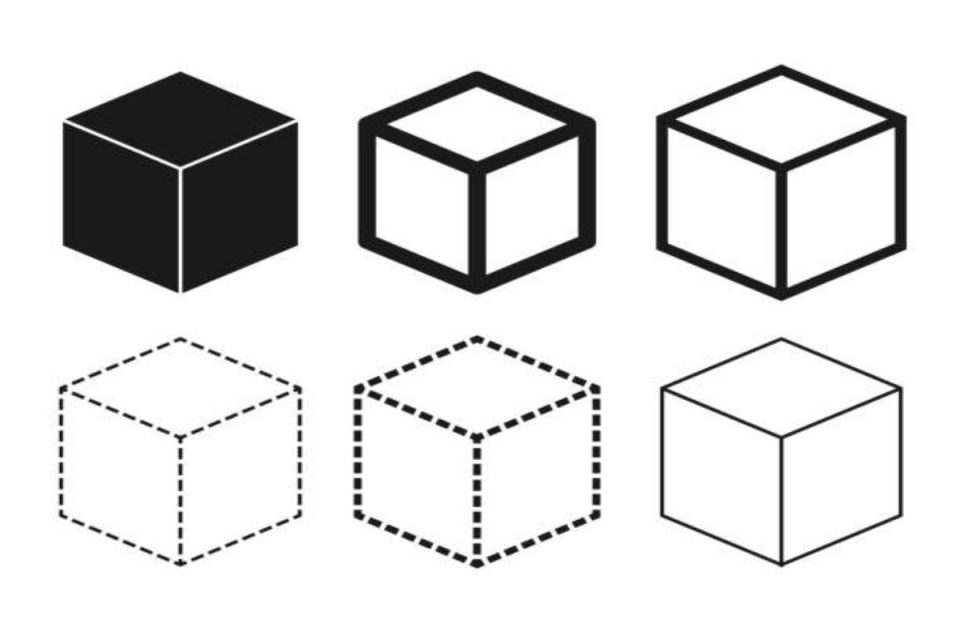General

# Square And Its FeaturesA square is a quadrilateral where all four sides are equal. Numerous types of square objects come across you in your daily life. Coming to the square it is characterized by one single dimension which is the side length. Let us understand about square in detail before we go ahead.

## Definition of square and their properties

It works out to be a two-dimensional figure where it has four sides along with four corners. The length of all the four sides would be equal and at the same time parallel to each other. It is a quadrilateral due to the following reasons

• All four sides are equal
• The angles measure 90 degrees
• The opposite sides work out to be parallel

Now let us understand in detail its properties. Normally it is a closed figure of equal four sides which is 90 degrees.  It is known to possess a wide range of properties. The important properties of a square are as follows

• It is a quadrilateral with four equal sides and four vertices
• Its four sides  are equal to each other
• The opposite sides are parallel to each other.
• An interior angle of it is a vertex at 90 degrees
• The sum of all interior angles is 360 degrees
• Even  its length of the diagonals is equal
• As its sides are parallel it is also referred to as a parallelogram
• The diagonals length in it would be greater than its sides
• The diagonals of a square are known to divide into two concurrent triangles
• The length of its diagonals  would be greater than its sides

In addition, there are some common properties associated with it and rectangles. Below are mentioned some common properties of a square and a rectangle that defines them

• Both of them are quadrilateral with four equal sides and equal vertices
• The opposite sides of the rectangle and square are parallel to each other
• The interior angle of a rectangle and it has a vertex at 90 degrees
• Both its diagonals and rectangle divides it into two equal halves
• It is also known as a parallelogram

## The formulas of the square

All of us are aware that it is an equal-shaped figure where all four sides are equal. Three basic types of formulas are there that we tend to use in geometry. The first one would be to calculate area of square formula, then we need to derive the perimeter and the last is going to be the design of the its formula.

Coming to the area of a square it is the side enclosed inside the square. There are numerous examples of it that we come across in our daily life like a chessboard, and we arrive at the formula by s2 units. Once the diagonal of the squared is known, it becomes easy to calculate the formula of the square

The perimeter of it would be the length that is present outside the shape of it. You arrive at the perimeter by adding up the length of all four sides. As it is known to have four sides we need to add up all of them to arrive at the perimeter

Coming to the best place where you may obtain information about square it is Cuemath. It is one of the best platforms to educate you on the topic.  They have some eminent professionals to address all your queries.